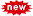# Promotion Lists 2022-23 | Calculation of Marks

Promotion Lists 2022-23 Promotion Lists PDF 2022-23 Promotion Lists Abstract Prmotion Lists Class 1-9 th Class Abstract

Promotion Lists 2022-23 | Calculation of Marks

## Class wise Calculation of marks for promotion list

Calculation for 3rd to 5th Classes: (FA 1)+(FA 2)+(FA 3)+(FA 4)+(SA 1)=50+50+50+50+50 = 250 M Reduced to 50 Marks and SA2 for 50 Marks, Total for 100M.

Calculation for 6th, 7th & 8th Classes: (FA 1)+(FA 2)+(FA 3)+(FA 4)+(SA 1)= 50+50+50+50+80=280 M Reduced to 20 Marks and SA2 for 80 Marks, Total for 100M.

Calculation for 8th Class PS: (FA 1)+(FA 2)+(FA 3)+(FA 4)+(SA 1)= 50+50+50+50+40=240 M Reduced to 10 Marks and SA2 for 40 Marks, Total for 50M.

Calculation for 8th Class BS: (FA 1)+(FA 2)+(FA 3)+(FA 4)+(SA 1)= 50+50+50+50+40=240 M Reduced to 10 Marks and SA2 for 40 Marks, Total for 50M.

Calculation for 9th Classes: (FA 1)+(FA 2)+(FA 3)+(FA 4)+(SA 1)= 50+50+50+50+100 = 300 M Reduced to 20 Marks and SA2 for 100 Marks, it is reduced to 80 Marks, Total for 100M.

Calculation for 9th Class for PS: (FA 1)+(FA 2)+(FA 3)+(FA 4)+(SA 1)=50+50+50+50+50 = 250 M Reduced to 10 Marks and SA2 for 50 Marks, it is reduced to 40Marks, Total for 50M.

Calculation for 9th Class for BS: (FA 1)+(FA 2)+(FA 3)+(FA 4)+(SA 1)=50+50+50+50+50 = 250 M Reduced to 10 Marks and SA2 for 50 Marks, it is reduced to 40Marks, Total for 50M.

Posted in: ,

## AP Latest InformationLearn a Word September 2022 ScheduleMore

More

More
Top